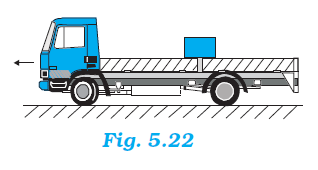## Pages

### Laws of motion NCERT Solutions Class 11 Physics - Solved Exercise Question 5.36

Question 5.36:
The rear side of a truck is open and a box of 40 kg mass is placed 5 m away from the open end as shown in Fig. 5.22. The coefficient of friction between the box and the surface below it is 0.15. On a straight road, the truck starts from rest and accelerates with 2 m s–2. At what distance from the starting point does the box fall off the truck? (Ignore the size of the box).Solution:
Mass of the box, m = 40 kg
Coefficient of friction, μ = 0.15
Initial velocity, u = 0
Acceleration, a = 2 m/s2
Distance of the box from the end of the truck, s' = 5 m
As per Newton’s second law of motion, the force on the box caused by the accelerated motion of the truck is given by:
F = ma
= 40 × 2 = 80 N
As per Newton’s third law of motion, a reaction force of 80 N is acting on the box in the backward direction. The backward motion of the box is opposed by the force of friction f, acting between the box and the floor of the truck. This force is given by:
f = μmg
= 0.15 × 40 × 10 = 60 N
∴Net force acting on the block:
Fnet = 80 – 60 = 20 N backward
The backward acceleration produced in the box is given by:
aback = Fnet / m  =  20 / 40 = 0.5 ms-2
Using the second equation of motion, time t can be calculated as:
s' = ut + (1/2)abackt2
5 = 0 + (1/2) X 0.5 X t2
∴ t = √20 s
Hence, the box will fall from the truck after √20 s from start.
The distance s, travelled by the truck in √20 s is given by the relation:
s = ut + (1/2)at2
= 0 + (1/2) X 2 X (√20)2
= 20 m